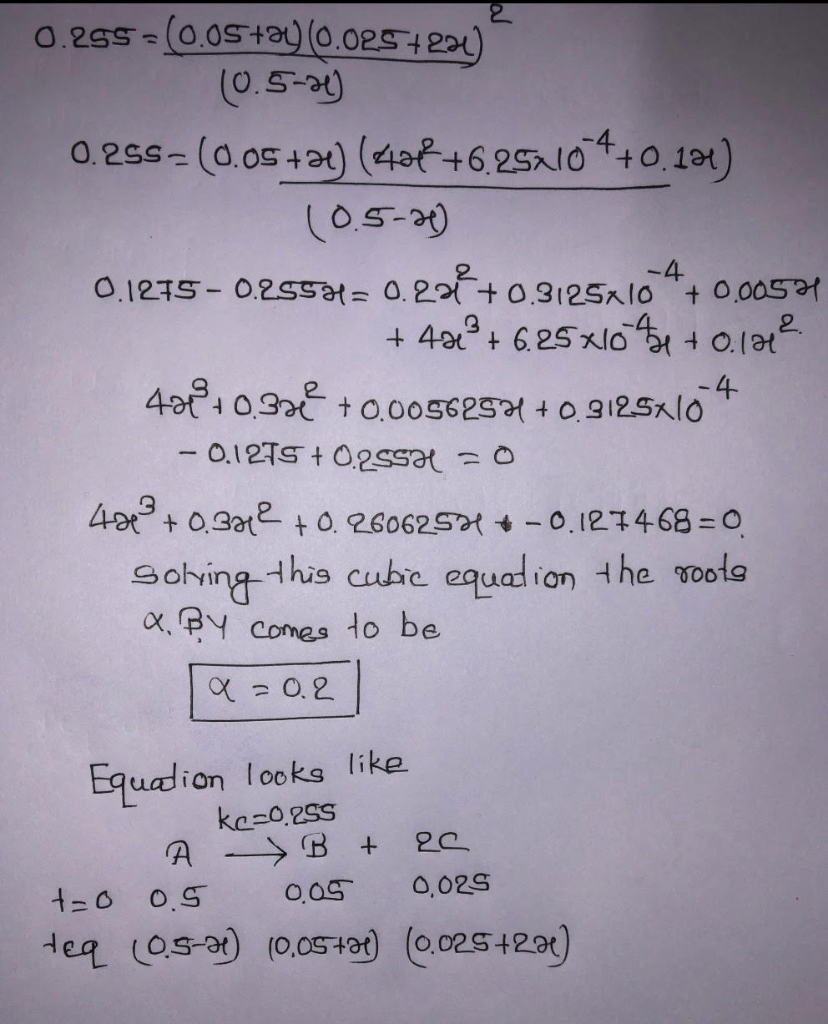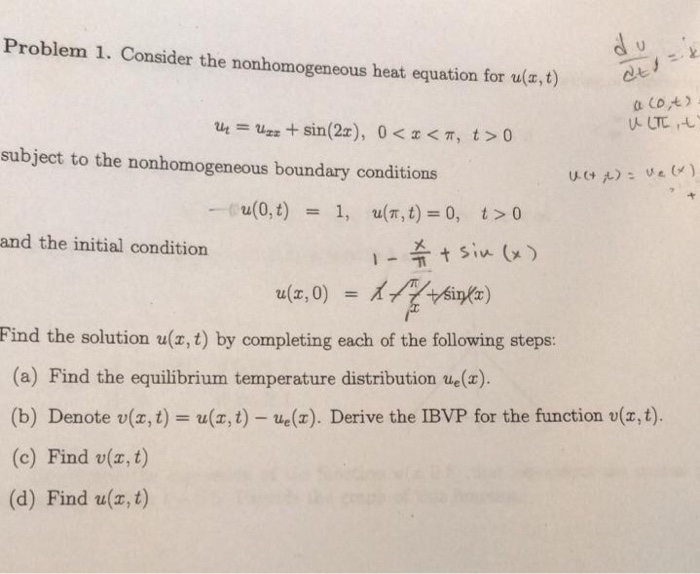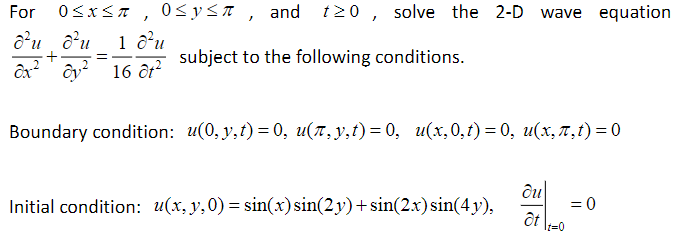Question

# For the following equilibrium expression: (0.05+x)(0.025+2x)^2 / (0.5-x)=0.255 Find the boundary conditions and roots for this...

For the following equilibrium expression:

(0.05+x)(0.025+2x)^2 / (0.5-x)=0.255

Find the boundary conditions and roots for this equation([-0.0125, 0.5)]

approximate solution is 0.2

In terms of A’s, B’s, C’s, etc. what do you suspect the reaction for this equation looks like?

please dont leave out any steps#### Earn Coins

Coins can be redeemed for fabulous gifts.

Similar Homework Help Questions
• ### Problem 1. Consider the nonhomogeneous heat equation for u,t) ut = uzz + sin(2x), 0<x<π, t>0 subject to the nonhomogeneous boundary conditions u(0, t) t > 0 u(n, t) = 0, 1, - and the...Problem 1. Consider the nonhomogeneous heat equation for u,t) ut = uzz + sin(2x), 0<x<π, t>0 subject to the nonhomogeneous boundary conditions u(0, t) t > 0 u(n, t) = 0, 1, - and the initial condition Lee) Find the solution u(z, t) by completing each of the following steps: (a) Find the equilibrium temperature distribution ue(x). (b) Denote v(x, t) u(a, t) - e(). Derive the IBVP for the function v(x,t). (c) Find v(x, t) (d) Find u(, t)...

• ### For 0 x π , 0S9, π , and 120 , solve the 2-D wave equation subject to the following conditions. u(0,y,t)-0, u(T.yt):0, u(x,0,) u(x,π, t) 0, 0 Boundary condition: C11 1 u(x),0)-sin(x)sin(2y) + sin(2x)...For 0 x π , 0S9, π , and 120 , solve the 2-D wave equation subject to the following conditions. u(0,y,t)-0, u(T.yt):0, u(x,0,) u(x,π, t) 0, 0 Boundary condition: C11 1 u(x),0)-sin(x)sin(2y) + sin(2x)sin(4y), 0 at It=0 Initial condition: For 0 x π , 0S9, π , and 120 , solve the 2-D wave equation subject to the following conditions. u(0,y,t)-0, u(T.yt):0, u(x,0,) u(x,π, t) 0, 0 Boundary condition: C11 1 u(x),0)-sin(x)sin(2y) + sin(2x)sin(4y), 0 at It=0 Initial condition: